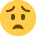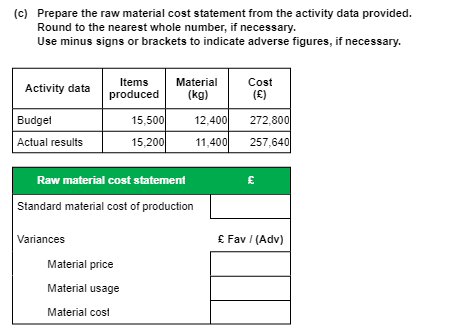# MABU - Assesment 2 Q6 C HELP!

Hi guys

I've been staring at this question for an hour now and still can't work it out...I'm sitting my exam in a few days and starting to freakAny ideas?

Thanks in advance!

## Comments

• Hi,
To find the standard cost of material take the budget cost and divide by budget units and then multiply by the actual units. (272800 / 15500 x 15200)
Material price is budget cost divided by material (kg) = std price per kg multiplied by actual material used (272800 / 12400 = £22 per std kg, £22 x 11400 = 250800) This is what the materials should have cost. Then just subtract the actual figure to get the variance (250800-257640 = an adverse variance of £6840.
Sign In or Register to comment.